# Star polyhedron

In geometry, a star polyhedron is a polyhedron which has some repetitive quality of nonconvexity giving it a star-like visual quality.

There are two general kinds of star polyhedron:

• Polyhedra which self-intersect in a repetitive way.
• Concave polyhedra of a particular kind which alternate convex and concave or saddle vertices in a repetitive way. Mathematically these figures are examples of star domains.

Mathematical studies of star polyhedra are usually concerned with regular, uniform polyhedra, or the duals of the uniform polyhedra. All these stars are of the self-intersecting kind.

## Self-intersecting star polyhedra

### Regular star polyhedra

The regular star polyhedra, are self-intersecting polyhedra. They may either have self-intersecting faces, or self-intersecting vertex figures.

There are four regular star polyhedra, known as the Kepler-Poinsot polyhedra. The Schläfli symbol {p,q} implies faces with p sides, and vertex figures with q sides. Two of them have pentagrammic {5/2} faces and two have pentagrammic vertex figures.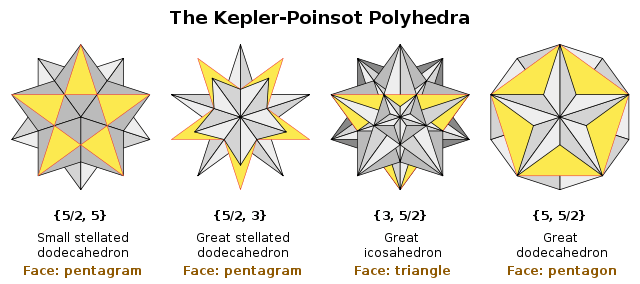These images show each form with a single face colored yellow to show the visible portion of that face.

### Uniform and uniform dual star polyhedra

There are many uniform star polyhedra including two infinite series, of prisms and of antiprisms, and their duals.

The uniform and dual uniform star polyhedra are also self-intersecting polyhedra. They may either have self-intersecting faces, or self-intersecting vertex figures or both.

The uniform star polyhedra have regular faces or regular star polygon faces. The dual uniform star polyhedra have regular faces or regular star polygon vertex figures.

Example uniform polyhedra and their duals
Uniform polyhedron Dual polyhedron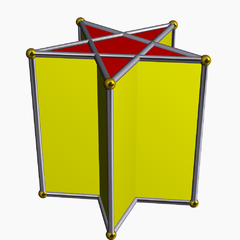The pentagrammic prism is a prismatic star polyhedron. It is composed of two pentagram faces connected by five intersecting square faces.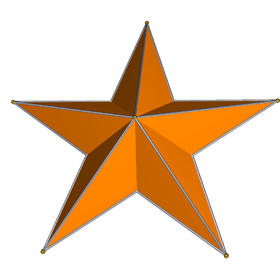The pentagrammic dipyramid is also a star polyhedron, representing the dual to the pentagrammic prism. It is face-transitive, composed of ten intersecting isosceles triangles.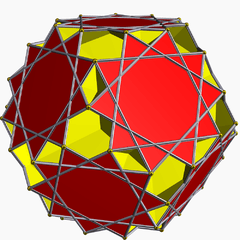The great dodecicosahedron is a star polyhedron, constructed from a single vertex figure of intersecting hexagonal and decagrammic, {10/3}, faces.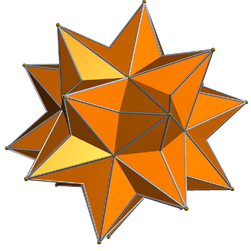The great dodecicosacron is the dual to the great dodecicosahedron. It is face-transitive, composed of 60 intersecting bow-tie-shaped quadrilateral faces.

### Stellations and facettings

Beyond the forms above, there are unlimited classes of self-intersecting (star) polyhedra.

Two important classes are the stellations of convex polyhedra and their duals, the facettings of the dual polyhedra.

For example, the complete stellation of the icosahedron (illustrated) can be interpreted as a self-intersecting polyhedron composed of 12 identical faces, each a (9/4) wound polygon. Below is an illustration of this polyhedron with one face drawn in yellow.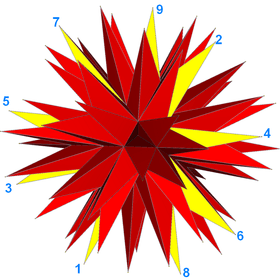### Star polytopes

A similarly self-intersecting polytopes in any number of dimensions is called a star polytope.

A regular polytope {p,q,r,...,s,t} is a star polytope if either its facet {p,q,...s} or its vertex figure {q,r,...,s,t} is a star polytope.

In four dimensions, the 10 regular star polychora are called the Schläfli-Hess polychora. Analogous to the regular star polyhedra, these 10 are all composed of facets which are either one of the five regular Platonic solids or one of the four regular star Kepler-Poinsot polyhedra.

For example, the great grand stellated 120-cell, projected orthogonally into 3-space, looks like this: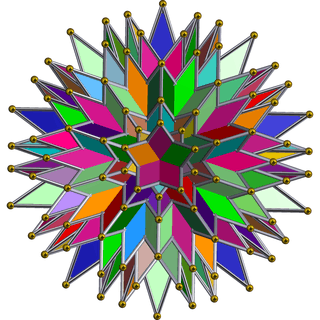There are no regular star polytopes in dimensions higher than 4.

## Star-domain star polyhedra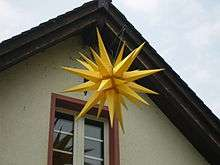A Moravian star hung outside a church

A polyhedron which does not cross inside itself, and all of the interior can be seen from one point, is an example of a star domain. Many star polyhedra are of this type (although star polyhedra and star domains have no formal mathematical relationship).

Such star polyhedra appear in various types of architecture, usually religious in nature. For example they are seen on many baroque churches as symbols of the Pope who built the church, on Hungarian churches and on other religious buildings. These stars can also be used as decorations. Moravian stars are used for both purposes and can be constructed in various forms.